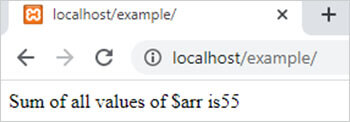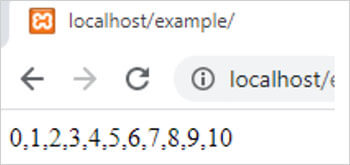array_reduce() function in php || user define function# array_reduce() function in php

1578

array_reduce() function of PHP is used to reduce a PHP array into a single variable with the help of a user define function or a callback function. It is an inbuilt function of PHP.

Syntax:

array_reduce(\$array,user_function,\$initial);

here,

• \$array is a PHP array. It is mandatory
• user_function is the user define function which has conditions to be applied on an array. It is also mandatory.
• \$initial is the value which send to the user define function. It is optional.

Example 1:

```<?php
// This example add all elements of array and return their addition
function user_function(\$x,\$y)
{
\$x = \$x + \$y;
return \$x;
}
\$arr = array(1,2,3,4,5,6,7,8,9,10);
\$result=array_reduce(\$arr,"user_function","0");
echo 'Sum of all values of \$arr is '.\$result;
?>```

Output:Example 2:

```<?php
// reduce all values of array into a single string separated by commas
function user_function(\$x,\$y)
{
\$x = \$x.','.\$y;
return \$x;
}
\$arr = array(1,2,3,4,5,6,7,8,9,10);
\$result=array_reduce(\$arr,"user_function","0");
echo \$result;
?>```

Output:Share:## Big Hosting Days

Upto 75@ OFF on Web Hosting

## Get a .COM for just \$6.98

Secure Domain for a Mini Price